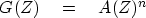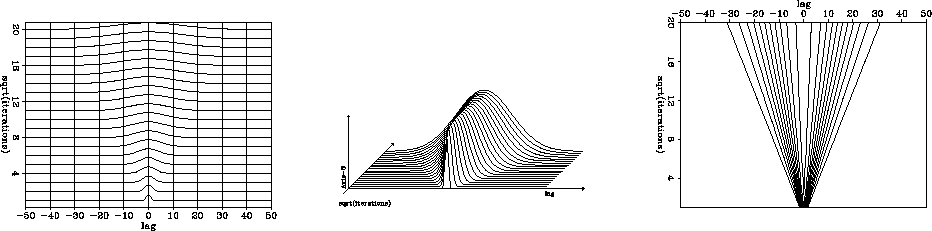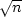# THE CENTRAL-LIMIT THEOREM

The central-limit theorem of probability and statistics is perhaps the most important theorem in these fields of study. A derivation of the theorem explains why the Gaussian probability function is so frequently encountered in nature; not just in physics but also in the biological and social sciences. No experimental scientist should be unaware of the basic ideas behind this theorem. Although the central-limit theorem is deep and is even today the topic of active research, we can quickly go to the basic idea.

From equation (59), if we add n random numbers, the probability that the sum of them equals k is given by the coefficient of Zk in(60)
The central-limit theorem says that as n goes to infinity, the polynomial G(Z) goes to a special form, almost regardless of the specific polynomial A(Z). The specific form is such that a graph of the coefficients of G(Z) comes closer and closer to fitting under the envelope of the bell-shaped Gaussian function. This happens because, if we raise any function to a high enough power, eventually all we can see is the highest value of the function and its immediate environment, i.e., the second derivative there. We already saw an example of this in Figure. Exceptions to the central-limit theorem arise (1) when there are multiple maxima of the same height, and (2) where the second derivative vanishes at the maximum.clim
Figure 13
Left: wiggle plot style. Middle: perspective. Right: contour.

Although the central-limit theorem tells us that a Gaussian function is the limit as,it does not say anything about how fast the limit is attained. To test this, I plotted the coefficients offor large values of n. This signal is made up of scaled binomial coefficients. To keep signals in a suitable amplitude scale, I multiplied them by.Figure 13 shows views of the coefficients of(horizontal axis) versus(vertical axis). We see that scaling byhas kept signal peak amplitudes constant. We see also that the width of the signal increases linearly with.The contours of constant amplitude show that the various orders 1